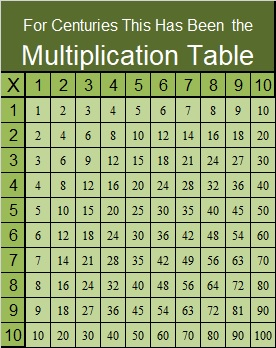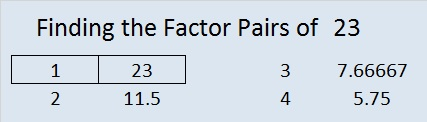# 23 You Call That a Multiplication Table? THIS is a Multiplication Table

Contents

### A Discussion About the Multiplication Table:

Almost everyone is familiar with the traditional multiplication table:Is this traditional way the best way for children to learn multiplication facts? Maybe not!

Beyondtraditionalmath published a post showing the Griddle’s creation of a different kind of multiplication table. This table can really help anyone visualize what multiplication means by relating any multiplication fact to its area. Every child learning to multiply should be exposed to this wonderful table. Even students who already know how to multiply would benefit from looking at it.

On the other hand, I hope it doesn’t completely replace the traditional multiplication table because that old, familiar table is the basis for my Find the Factor puzzles.

### Factors of the Number 23:

• 23 is a prime number.
• Prime factorization: 23 is prime.
• The exponent of prime number 23 is 1. Adding 1 to that exponent we get (1 + 1) = 2. Therefore 23 has exactly 2 factors.
• Factors of 23: 1, 23
• Factor pairs: 23 = 1 x 23
• 23 has no square factors that allow its square root to be simplified. √23 ≈ 4.79583How do we know that 23 is a prime number? If 23 were not a prime number, then it would be divisible by at least one prime number less than or equal to √23 ≈ 4.8. Since 23 cannot be divided evenly by 2 or 3, we know that 23 is a prime number.

23 is never a clue in the FIND THE FACTORS puzzles.

### More About the Number 23:

23 is the sum of three consecutive prime numbers:
5 + 7 + 11 = 23.

## 4 thoughts on “23 You Call That a Multiplication Table? THIS is a Multiplication Table”

1.wbhs62

•ivasallay

You’re welcome, and it was so easy to do!

2.Steve Morris

A simple but very clever idea!

•ivasallay

Indeed. We have to wonder why centuries went by before ANYBODY thought to display it this way.

This site uses Akismet to reduce spam. Learn how your comment data is processed.